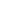# Ancient Mathematics: Greece

Ancient Greeks inherited mathematical knowledge from ancient Egyptian and Babylonians, but contrary to Egyptian scribes, they asked questions and searched for proof. From the 6th century BCE to the 4th century CE, from Thales and Pythagoras, to Aristotle and Euclid, and to Diophantus and a female ancient Greek mathematician Hypatia, they developed mathematics into deductive systematic science, filled with definitions, postulates, theorems, and proofs. The Greeks developed the theory of numbers, found all regular polyhedra, measured diameter of the Earth, proposed geocentric and heliocentric astronomical systems, and solved numerous theoretical and practical problems that became the basics of mathematics.

This class will be delivered online via the online platform Zoom. Enrolling students need to ensure they have an email, a reliable internet connection, microphone/speakers and access to a tablet, smartphone or computer.

• Science Awakening I: Egyptian, Babylonian and Greek Mathematics, B. L. Van Der Waerden. 2005, 4th Edition.

#### COURSE OUTLINE

• Main chronology and persons of ancient Greek mathematics.
• Greek numeral systems.
• Three great mathematical problems: doubling the cube; trisecting the angle; squaring the circle.
• Pythagorean number theory. Irrationality.
• Elements by Euclid.
• Archimedes: his mathematical discoveries, legends about him. Hypatia, a female mathematician.

#### PLANNED LEARNING OUTCOMES

By the end of this course, students should be able to:

1. Understand ancient Greek numeral systems.
2. Know main timeline of ancient Greek mathematics.
3. Know concepts of Pythagorean theory of harmony and theory of numbers.
4. Know Platonic regular polyhedra.
5. Become acquainted with axiomatic geometry by Euclid and Archimedes as a geometer and engineer.
\$65 Limited

<p>Ancient Greeks inherited mathematical knowledge from ancient Egyptian and Babylonians, but contrary to Egyptian scribes, they asked questions and searched for proof. From the 6th century BCE to the

...
11 Feb
Online Platform
Mo Tu We Th Fr Sa SuInterested in this course but can't attend? Please join the waiting list by clicking .